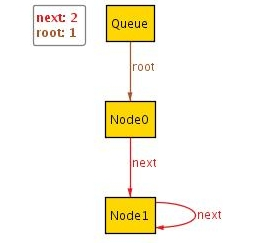A Guide to Alloy
 Home Introduction Tutorial Getting Started Statics I Statics II Dynamics I Dynamics II Alternatives to Alloy Test Yourself! Glossary FAQs Final Thoughts
Statics I
In this tutorial, we will be using Alloy to model a linked list implementation of a Queue: Every queue has a root node and each node has a reference to the next node in the queue.

Each Alloy model starts with the module declaration:
```	module examples/tutorial/Queue
```
This is similar to declaring a class Queue in the package examples.tutorial in Java. Similar to Java, the file should be named 'Queue.als' and be located in the subfolder examples/tutorial of the project folder.

The first step is to declare the signature for the queue and its nodes:
```	sig Queue { root: lone Node }
sig Node { next: lone Node }
```
You can think of root and next as being fields of type Node. lone is a multiplicity keyword which indicates that each Queue has less than or equal to one root (similarly for next).

A signature in Alloy is similar to the signature of a schema in that it defines the vocabulary for the model.

We can already visualize what we have written so far. The common way of doing this is to write a predicate and then make Alloy produce instances that satisfy this predicate. Asking Alloy to find instances is similar to finding a model of a given schema.

Because we want any instance of the Queue so far, the predicate has no constraints:
```	pred show() {}
```
To get sample instances of the predicate we use the command run:
```	run show for 2
```
A very important fact about Alloy is that it is only designed to search for instances within a finite scope. In the above case this scope is set to at most 2 objects in each signature(i.e. at most two Queues and at most two Nodes). Note that this is always an upper bound; Alloy may return a smaller instance.

In order to produce and visualize an instance, we first execute the run command by clicking on the Execute button. After the execution has finished, Alloy will tell us that it has found an instance which we can visualize by clicking on the Show button.
 ``` module examples/tutorial/Queue sig Queue { root: Node } sig Node { next: lone Node } pred show() {} run show for 2 ```In the above image, we see that Node1 is its own successor. As this is not what we usually expect from a linked list, we add a fact to constrain our model:
```	fact nextNotReflexive { no n:Node | n = n.next }
```
Facts in Alloy are 'global' in that they always apply and they correspond to the axioms of a schema. When Alloy is searching for instances it will discard any that violate the facts of the model. The constraint above can be read just like in first order logic: there is no Node n that is equal to its successor.

Executing run show now produces the following instance:
 ``` module examples/tutorial/Queue sig Queue { root: Node } sig Node { next: lone Node } fact nextNotReflexive { no n:Node | n = n.next } pred show() {} run show for 2 ```We no longer have a Node that is its own successor, but now notice another problem of our model: There are Nodes that do not belong to any Queue.